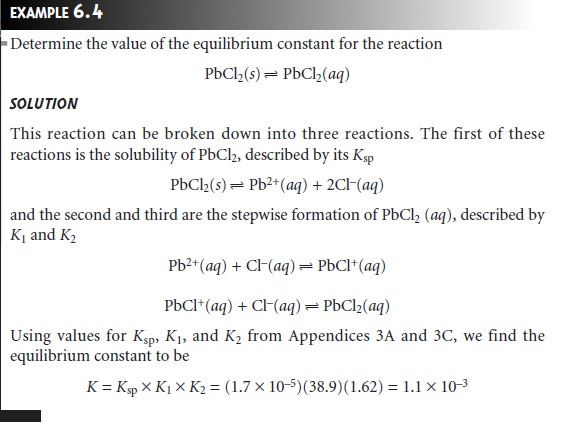Home | | Modern Analytical Chemistry | Equilibrium Constants for Complexation Reactions

# Equilibrium Constants for Complexation Reactions

A more general definition of acids and bases was proposed by G. N. Lewis (1875â€“1946) in 1923.

Complexation Reactions

A more general definition of acids and bases was proposed by G. N. Lewis (1875â€“1946) in 1923. The BrĂ¸nstedâ€“Lowry definition of acids and bases focuses on an acidâ€™s proton-donating ability and a baseâ€™s proton-accepting ability. Lewis the- ory, on the other hand, uses the breaking and forming of covalent bonds to describe acidâ€“base characteristics. In this treatment, an acid is an electron pair acceptor, and a base is an electron pair donor. Although Lewis theory can be applied to the treat- ment of acidâ€“base reactions, it is more useful for treating complexation reactions between metal ions and ligands.

The following reaction between the metal ion Cd2+ and the ligand NH3 is typical of a complexation reaction.The product of this reaction is called a metalâ€“ligand complex. In writing the equa- tion for this reaction, we have shown ammonia as :NH3 to emphasize the pair of electrons it donates to Cd2+. In subsequent reactions we will omit this notation.

The formation of a metalâ€“ligand complex is described by a formation con- stant, Kf. The complexation reaction between Cd2+ and NH3, for example, has the following equilibrium constantThe reverse of reaction 6.15 is called a dissociation reaction and is characterized by a dissociation constant, Kd, which is the reciprocal of Kf.

Many complexation reactions occur in a stepwise fashion. For example, the re- action between Cd2+ and NH3 involves four successive reactionsThis creates a problem since it no longer is clear what reaction is described by a for- mation constant. To avoid ambiguity, formation constants are divided into two cat- egories. Stepwise formation constants, which are designated as Ki for the ith step, describe the successive addition of a ligand to the metalâ€“ligand complex formed in the previous step. Thus, the equilibrium constants for reactions 6.17â€“6.20 are, re- spectively, K1, K2, K3, and K4. Overall, or cumulative formation constants, which are designated as Î˛i, describe the addition of i ligands to the free metal ion. The equilibrium constant expression given in equation 6.16, therefore, is correctly iden- tified as Î˛4, whereStepwise and cumulative formation constants for selected metalâ€“ligand complexes are given in Appendix 3C.

Equilibrium constants for complexation reactions involving solids are defined by combining appropriate Ksp and Kf expressions. For example, the solubility of AgCl increases in the presence of excess chloride as the result of the following com- plexation reactionThis reaction can be separated into three reactions for which equilibrium constants are knownâ€”the solubility of AgCl, described by its KspThe equilibrium constant for reaction 6.21, therefore, is equal to Ksp x K1 x K2Study Material, Lecturing Notes, Assignment, Reference, Wiki description explanation, brief detail
Modern Analytical Chemistry: Equilibrium Chemistry : Equilibrium Constants for Complexation Reactions |